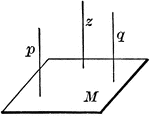### Parallel Lines

"Two straight lines that are parallel to a third are parallel to each other."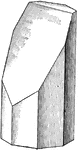### Plane Intersecting Octagonal Prism

Illustration showing the intersection of a plane with an octagonal prism.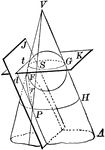### Cone Intersected by a Plane to Form a Parabola

Diagram of a cone intersected by plane J to form a parabola. Also pictured is a circle formed by the…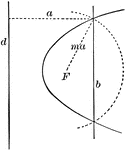### Construction of a Parabola

Diagram showing how to construct a parabola when given the directrix (d), the focus (F) and the eccentricity…### Front View Pusher Aeroplane Plan

A pusher aeroplane plan illustrating the proper ribbing and wiring for the plane. The wires are used…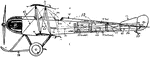### Propeller Aeroplane Side Plan

A propeller airplane plan illustrating the proper location of the skeleton and placement of the wires…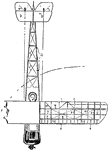### Propeller Aeroplane Top Plan

A top view plan of a propeller aeroplane, or airplane. The plan shows the proper ribs and skeleton for…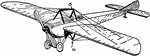### Propeller Aeroplane Top Plan

A side view plan of a propeller aeroplane for two people. The plan illustrates the the proper location…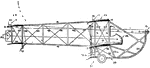### Pusher Aeroplane Plan

A plan for pusher aeroplane, or airplanes. The propeller of the pusher plane is located behind the cockpit…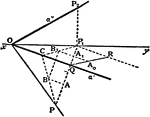### Angle of Two Planes

To determine the angle between two planes.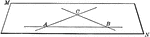### Plane Determined by 2 Intersecting Lines

Illustration of a plane determined by 2 intersecting lines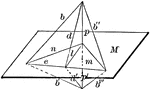### Line Perpendicular To Plane

"If a straight line is perpendicular to each of two straight lines at their point of intersection it…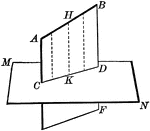### Line Projected Upon Plane

Illustration of a straight line projected upon a plane.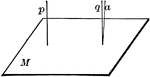### Lines Perpendicular To A Plane

"If one of two parallels is perpendicular to a plane, the other is also."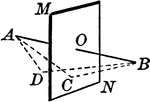### Locus Point to a Plane

"The locus of a point in space equidistant from the extremities of a straight line is the plane perpendicular…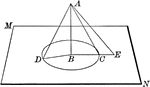### Oblique Lines Drawn to a Plane

Oblique lines drawn from a point to a plane.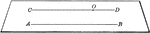### Plane Determined by 2 Parallel Lines

Illustration of a plane determined by 2 parallel lines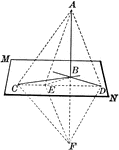### Line Perpendicular to Plane

If a straight line is perpendicular to each of two other straight lines in a plane at their point of…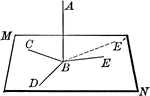### Line Perpendicular to Plane

Straight line AB is perpendicular to the lines in plane MN at point B.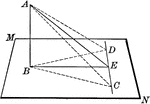### Line Perpendicular to Plane

Straight line AB is perpendicular to the lines in plane MN at point B. "If from the foot of a perpendicular…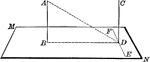### Lines Perpendicular to Plane

Two straight lines, AB and CD, perpendicular to the same plane (MN) are parallel.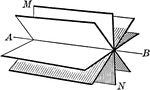### Plane Rotated About a Straight Line

Illustration of a plane rotated about a straight line.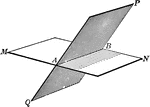### Intersecting Planes

The intersection of two planes is a straight line.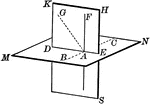### Intersecting Planes

The intersection of two planes is a straight line.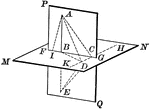### Intersecting Planes

The intersection of two planes is a straight line.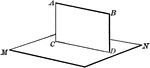### Intersecting Planes

The intersection of two planes is a straight line.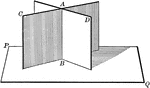### Intersecting Planes

Two intersecting planes on a third plane. "If two intersecting planes are each perpendicular to a third…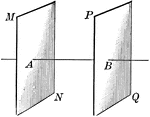### Parallel Planes

Illustration of two parallel planes. "Two planes perpendicular to the same straight line are parallel."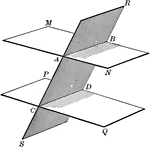### Parallel Planes

Two parallel planes cut by a third plane.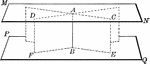### Parallel Planes

Two parallel planes perpendicular to a line (AB). "A straight line perpendicular to one of two parallel…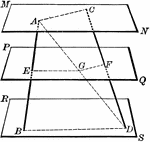### Parallel Planes Cut By 2 Lines

Three parallel planes cut by two lines.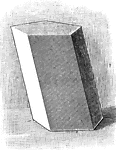### Prism

Illustration of a prism - a polyhedron of which two faces are equal polygons in parallel planes, and…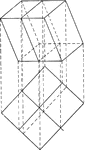### Intersection of a Prism and a Plane

Illustration of the intersection of a prism and a plane.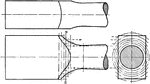### Intersection of Surface of Revolution and Plane

"A series of planes as S—S are passed perpendicular to the axis of revolution, cutting out the…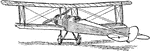### Airplane Rudder and Elevator

A propeller airplane with rudder, elevator, and ailerons. The rudder stabilizes the plane vertical,…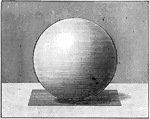### Sphere With Tangent Plane

Illustration of a sphere with a tangent plane. "A plane perpendicular to a radius at its extremity is…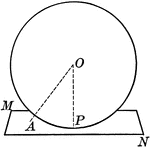### Sphere With Tangent Plane

Illustration of a sphere with a tangent plane. "A plane perpendicular to a radius at its extremity is…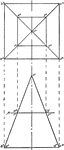### Intersection of Square Pyramid and a Plane

Illustration of the intersection of a square pyramid and a plane.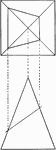### Intersection of Square Pyramid and a Plane

Illustration of the intersection of a square pyramid and a plane.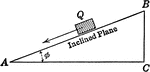### Inclined Plane Forming Right Triangle

Inclined plane forming right triangle showing the velocity of a body sliding a distance,s, down a smooth…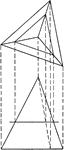### Intersection of Triangular Pyramid and a Plane

Illustration of the intersection of a triangular pyramid and a plane.